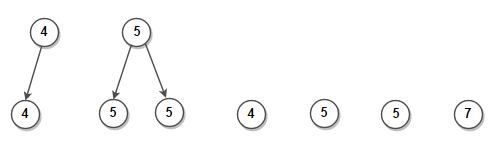# Count all subtrees having same value of nodes in a binary tree

Given a binary tree, count all subtrees in it such that every node in the subtree have same value.

For example, consider below treeThere are 6 subtrees having same dataA simple solution would be to consider every node and check if all nodes present in the subtree rooted at current node have same values or not. The time complexity of this solution is O(n2).

We can solve this problem in linear time. The idea is traverse the tree in postorder fashion. Then by comparing return values of left subtree and right subtree, we can easily check if subtree rooted at any node have same values or not.

C++ implementation –

The time complexity of above solution is O(n) and auxiliary space used by the program is O(1).(1 votes, average: 5.00 out of 5)Loading...

Please use our online compiler to post code in comments. To contribute, get in touch with us.
Like us? Please spread the word and help us grow. Happy coding 🙂Subscribe
Notify ofGuest

can this be done without using INT_MIN.. what if the node’s data is INT_MINGuest

You use LONG_MIN.Guest

I Can’t understand the example, how is 7 a part of the subtree having same data..?